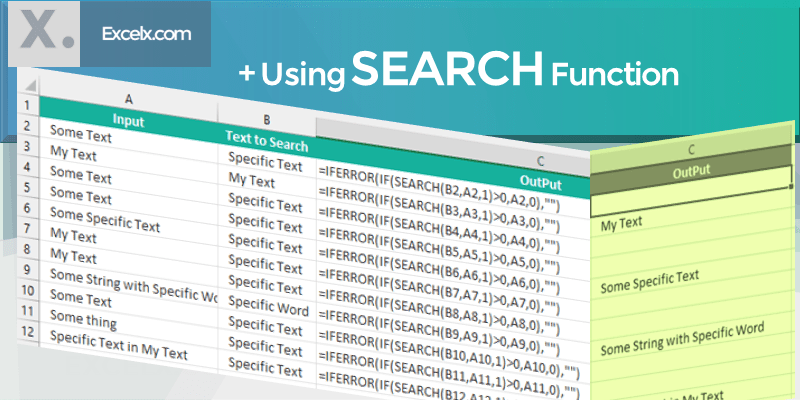# If Cell Contains Specific Text Then Return Value

Here is the the Excel formula to check if cell contains specific text then return value. Often, we need to search for a text in a cell or range of cells in the Excel, return if it contains a specific text or string. Following is the simple formula to find specific text in given cells and return its values.

## If Cell Contains Specific Text Then Return Value

Following is the Excel formula to find If Cell Contains Specific Text Then Return Value. This example used IF and COUNTIF functions to check for a value in a Cell and return required value.

`=IF(COUNTIF(A1,"*Specific Text*"),"Yes","No")`

You can see that we are counting the cells with specific text and returning the Value ‘Yes’ if found, ‘No’ if not found in the Cell.

### If Cell Contains Specific Text Then Return Value – Using COUNTIF Function

We can return the Cell value if using the same functions. You can also change the Specific Text to a Cell reference if you wants to make the function more dynamic.

`=IF(COUNTIF(A1,"*"&B2&"*"),A1,"")`

the above function returns the Cell Value if a specific text found in the Cell, otherwise it returns Blank.

See also  MicroLED watches, OLED MacBooks, touchscreen Macs...is Apple really going to do this stuff?

### If Cell Contains Specific Text Then Return Value – Using SEARCH Function

You can also achieve this by using Search Function. Below is the Excel formula to find If Cell Contains Specific Text Then Return Value. In our example, we have input data in Cell A2:A12 and We will Return the Values in Range B2:B12.

Here the Excel formula to Return Value If Cell Contains Specific Text :

`=IFERROR(IF(SEARCH(B2,A2,1)>0,A2,0),"")`

Values Passed in the SEARCH Function

• Parameter 1: B2=”find_text”, the text where you can replace with the specific text to be searched in another text
• Parameter 2: A2=”within_text”, where you will search for ‘find_text’
• Parameter 3: 1=Character number which you want start you search in within_text from the left.### Formula to Determine If Cell Contains Specific Text

Here is the simple formula to check if cell contains specific text or not. You can search for a text and return the required values (for example: Yes, No), if finding text existing in within cell.

`=IF(COUNTIF(A2,"*Specific Text*"),"Yes, it is Found", "Not Found")`

Here COUNTIF function determines if a specific text exist in a Cell. And the IF function return “Yes” if Found.

### Formula to Determine If Cell Contains Certain Text

You can use the following formula to Determine If Cell Contains Certain Text. You can change any text in the Cell B1 and you can see the result instantly.

`=IF(COUNTIF(A1,"*Certain Text*"),"TRUE","FALSE")`

We can make this more dynamic by passing certain text using a cell as shown below:

`=IF(COUNTIF(A1,"*" &B1 &"*"),"TRUE","FALSE")`

### Excel VBA If Cell Contains Certain Text

Here is the Excel VBA to check if Cell contains certain text. You can change the cell reference to any cell and specificText to any text.
`Sub MacroToCheckIfCellContainsSpecificText_vba()`

Set Cell = Cell(1, 4) ‘ You can change this
specificText = “Specific Text” ‘ You can change this

See also  Strictly Come Dancing: Bruno Tonioli praises new judge Anton Du Beke

If UCase(Cell.Value) Like “*” & UCase(specificText) & “*” Then
MsgBox “Found”
Else